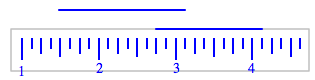### Home > MC1 > Chapter 8 > Lesson 8.1.1 > Problem8-10

8-10.

Katie is measuring two line segments. One extends from the $2\frac{3}{4}$-inch mark on her ruler to the $4\frac{1}{8}$-inch mark. The other extends from the $1\frac{1}{2}$-inch mark on the ruler to the $3\frac{1}{8}$-inch mark. How long is each piece? Which piece is longer? How much longer is it?The visualization above might be helpful.
If the line segments are too close to the same size to easily see which is longer, try using subtraction.